## Complete Functions

A set of Orthonormal Functions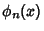is termed complete in the Closed Interval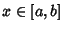if, for every piecewise Continuous Function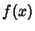in the interval, the minimum square error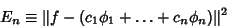(where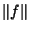denotes the Norm) converges to zero asbecomes infinite. Symbolically, a set of functions is complete if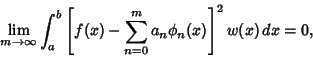where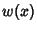is a Weighting Function and the above is a Lebesgue Integral.

Arfken, G. Completeness of Eigenfunctions.'' §9.4 in Mathematical Methods for Physicists, 3rd ed. Orlando, FL: Academic Press, pp. 523-538, 1985.